Subsections

# 3.1: A Singly-Linked List

An(singly-linked list) is a sequence of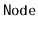s. Each node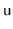stores a data value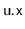and a reference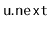to the next node in the sequence. For the last node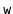in the sequence,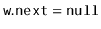```  class Node {
public:
T x;
Node *next;
Node(T x0) {
x = 0;
next = NULL;
}
};
```

For efficiency, an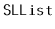uses variablesand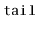to keep track of the first and last node in the sequence, as well as an integer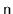to keep track of the length of the sequence:

```  Node *head;
Node *tail;
int n;
```
A sequence of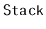andoperations on anis illustrated in Figure 3.1.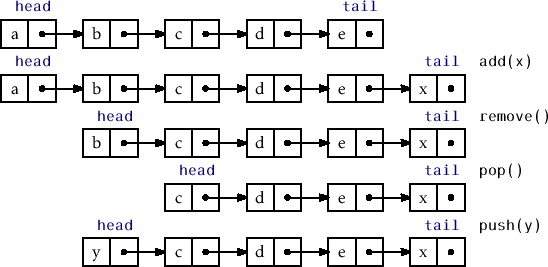Ancan efficiently implement theoperations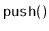andby adding and removing elements at the head of the sequence. Theoperation simply creates a new nodewith data value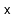, setsto the old head of the list and makesthe new head of the list. Finally, it incrementssince the size of thehas increased by one:

```  T push(T x) {
Node *u = new Node(x);
if (n == 0)
tail = u;
n++;
return x;
}
```

The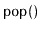operation, after checking that theis not empty, removes the head by setting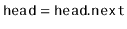and decrementing. A special case occurs when the last element is being removed, in which caseis set to:

```  T pop() {
if (n == 0)  return null;
delete u;
if (--n == 0) tail = NULL;
return x;
}
```

Clearly, both theandoperations run intime.

## 3.1.1 Queue Operations

Ancan also implement the FIFO queue operationsand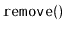in constant time. Removals are done from the head of the list, and are identical to theoperation:

```  T remove() {
if (n == 0)  return null;
delete u;
if (--n == 0) tail = NULL;
return x;
}
```

Additions, on the other hand, are done at the tail of the list. In most cases, this is done by setting, whereis the newly created node that contains. However, a special case occurs when, in which case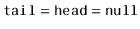. In this case, bothand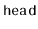are set to.

```  bool add(T x) {
Node *u = new Node(x);
if (n == 0) {
} else {
tail->next = u;
}
tail = u;
n++;
return true;
}
```

Clearly, both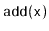andtake constant time.

## 3.1.2 Summary

The following theorem summarizes the performance of an:

Theorem 3..1   Animplements theand (FIFO)interfaces. The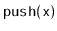,,andoperations run intime per operation.

Annearly implements the full set ofoperations. The only missing operation is removing from the tail of an. Removing from the tail of anis difficult because it requires updating the value ofso that it points to the nodethat precedesin the; this is the nodesuch that. Unfortunately, the only way to get tois by traversing thestarting atand takingsteps.

opendatastructures.org